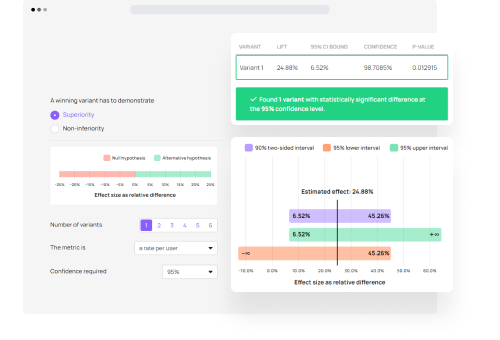A/B testing

# Statistical significance calculator

P-value and confidence interval calculator for analysis of online A/B tests.

Use all our tools for 30 days! No credit card required.## Features of the A/B test calculator

• Calculates statistical significance (p-values) and confidence intervals
• Works with both binomial and non-binomial metrics
• Analyze tests with multiple variants (A/B/N tests)
• Supports non-inferiority test designs
• Outputs statistics for percent change (lift)
• Trustworthy outcomes due to in-built SRM checker
• Excellent documentation and presentation of calculation results

## Statistical significance calculations done right## Support for non-binomial metrics

The significance calculator supports the calculation of non-binomial (continuous) metrics such as average revenue per user, average sessions per user, average session duration, average order value, etc. out of the box. A user-friendly interface allows you to upload a file with your non-binomial data and all relevant statistics are calculated from it.

Obviously, it also supports the classic binomial metrics which are all kinds of conversion rates, dropout rates, etc.

## Properly analyze A/B/N tests

Tests with more than one variant versus a control need to be analyzed with special methods that account for the multiple comparisons problem that otherwise arises. This calculator uses a p-value and confidence interval correction known as Dunnett's correction which is automatically applied to all A/B/n test data you enter in the tool.## Analyze non-inferiority tests

If you have planned a test with a non-inferiority hypothesis it needs to be analyzed as such. This significance calculator has native support for non-inferiority tests.

Simply enter the non-inferiority margin used when planning the test and the tool will take care of the rest.

## Peace of mind checks

To help ensure trustworthy test outcomes, the data is checked for sample ratio mismatch under the assumption of equal allocation between test groups. Equal allocation is usually preferred since it is typically the most efficient method to conduct a test.## Excellent documentation

The calculator is documented in detail, with information about the statistical tests used and the assumptions applied shown below each computation. Inline help information assists in using the tool correctly and makes sure the statistical jargon, to the extent that it is unavoidable, is easy to navigate.### Take your A/B testing to the next level

Experience the power and utility of our A/B testing hub and all tools during our 30-day free trial. No credit card required.

### See what our clients have to say

A tool with real value if you know how to use it. Might seem intimidating at the beginning, but it tries to provide flexibility, accuracy and optimization in your statistical analysis instead of over-simplification.Arnau VendrellRyan Lucht